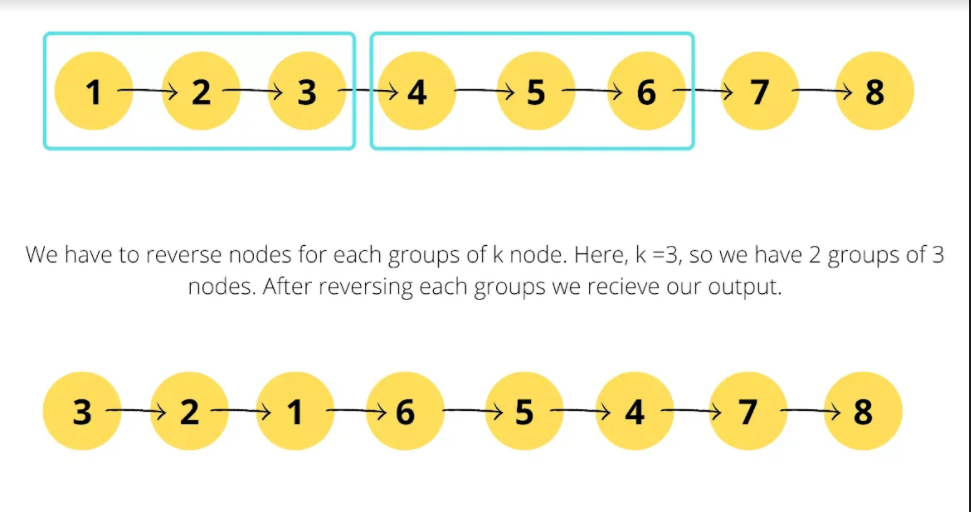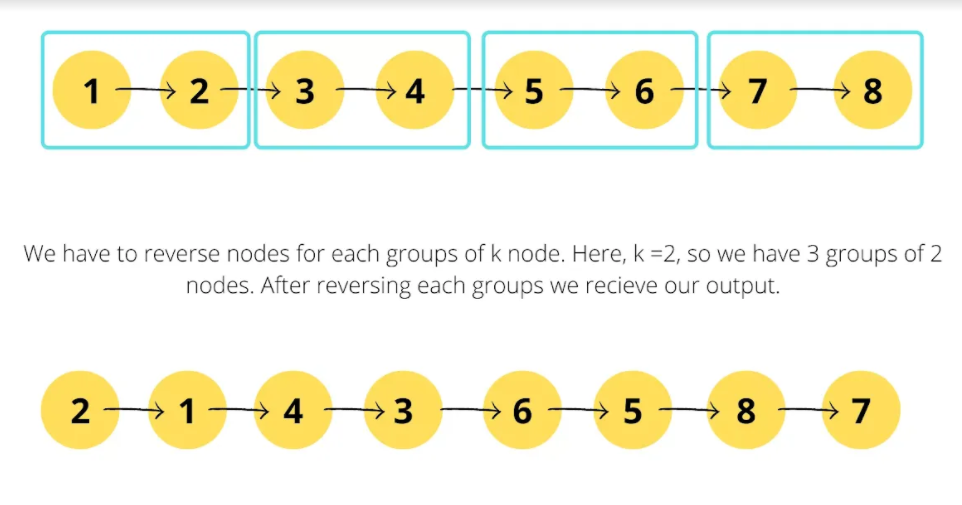# Reverse Linked List in groups of Size K

In this article, we will solve a very popular question Reverse Linked List in groups of Size K.

Problem Statement: Given the head of a linked list, reverse the nodes of the list k at a time, and return the modified list. k is a positive integer and is less than or equal to the length of the linked list. If the number of nodes is not a multiple of k then left-out nodes, in the end, should remain as it is.

Examples:

```Example 1:
Input:
Output:
Explanation:```
```Example 2:
Input:
Output:
Explanation:```

Solution:

Approach:

The following steps are needed to arrive at the desired output.

• Create a dummy node. Point next to this node to head of the linked list provided.
• Iterate through the given linked list to get the length of the list.
• Create three pointer pre,cur and nex to reverse each group. Why? If we observe output, we can see that we have to reverse each group, apart from modifying links of group.
• Iterate through the linked list until the length of list to be processed is greater than and equal to given k.
• For each iteration, point cur to pre->next and nex to nex->next.
• Start nested iteration for length of k.
• For each iteration, modify links as following steps:-
• cur->next = nex->next
• nex->next = pre->next
• pre->next = nex
• nex = cur->next
• Move pre to cur and reduce length by k.

Dry Run:

Code:

## C++ Code

``````#include<bits/stdc++.h>
using namespace std;

class node {
public:
int num;
node* next;
node(int a) {
num = a;
next = NULL;
}
};
//utility function to insert node in the list
node* newNode = new node(val);
return;
}

while(temp->next != NULL) temp = temp->next;

temp->next = newNode;
return;
}
//utility function to find length of the list
int length = 0;
++length;
}
return length;
}
//utility function to reverse k nodes in the list

node* cur;
node* nex;

while(length >= k) {
cur = pre->next;
nex = cur->next;
for(int i=1;i<k;i++) {
cur->next = nex->next;
nex->next = pre->next;
pre->next = nex;
nex = cur->next;
}
pre = cur;
length -= k;
}
}
//utility function to print the list
}
}

int main() {
int k = 3;

cout<<"After Reversal of k nodes: ";

return 0;
}
``````

Output:

After Reversal of k nodes: 3->2->1->6->5->4->7->8

Time Complexity: O(N)

Reason: Nested iteration with O((N/k)*k) which makes it equal to O(N).

Space Complexity: O(1)

Reason: No extra data structures are used for computation.

## Java Code

``````import java.util.*;
class Node {
int num;
Node next;
Node(int a) {
num = a;
next = null;
}
}
class TUF{
//utility function to insert node in the list
static Node insertNode(Node head,int val) {
Node newNode = new Node(val);
}

while(temp.next != null) temp = temp.next;

temp.next = newNode;
}
//utility function to find length of the list
int length = 0;
++length;
}
return length;
}
//utility function to reverse k nodes in the list
static Node reverseKNodes(Node head,int k) {

Node cur;
Node nex;

while(length >= k) {
cur = pre.next;
nex = cur.next;
for(int i=1;i<k;i++) {
cur.next = nex.next;
nex.next = pre.next;
pre.next = nex;
nex = cur.next;
}
pre = cur;
length -= k;
}
}
//utility function to print the list
}
}

public static void main(String args[]) {
int k = 3;

System.out.print("After Reversal of k nodes: ");

}
}
``````

Output:

After Reversal of k nodes: 3->2->1->6->5->4->7->8

Time Complexity: O(N)

Reason: Nested iteration with O((N/k)*k) which makes it equal to O(N).

Space Complexity: O(1)

Reason: No extra data structures are used for computation.

## Python Code

``````class Node:
def __init__(self, val):
self.val = val
self.next = None

# utility function to insert node at the end of the linked list
newNode = Node(val)
while temp.next != None:
temp = temp.next
temp.next = newNode

# utility function to find length of the linked list
length = 0
length += 1
return length

# utility function to reverse k nodes in the linked list

cur = None
nex = None

while length >= k:
cur = pre.next
nex = cur.next
for i in range(1, k):
cur.next = nex.next
nex.next = pre.next
pre.next = nex
nex = cur.next
pre = cur
length -= k

# utility function to print the linked list

if __name__ == "__main__":
k = 3

print("After Reversal of k nodes: ", end="")

Output: